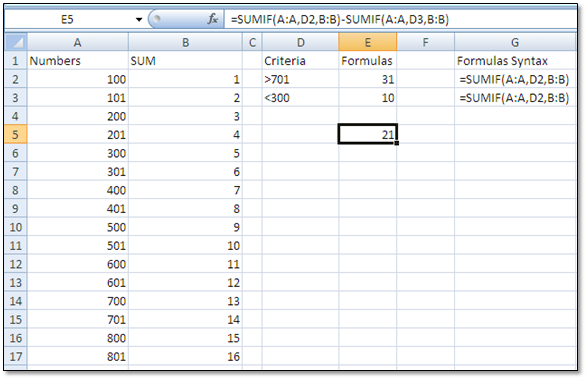## NIFTY TIP Adding or Subtracting Amounts from Different Ranges

Problem: You need to add or subtract amounts from different ranges according to a specific criteria (SUMIF function).

Solution: Insert two SUMIF formulas and combine them into a single formula:

1. Insert the SUMIF formula to total the amounts based on the criterion 701 into cell E2.
2. Insert the SUMIF formula to total the amounts based on the criterion 300 into cell E3.
3. Select cell E2 and copy the formula from the Formula Bar, select the formula and press <ctrl>+C and click the Enter or Cancel symbol in the Formula Bar to exit Edit mode.
4. Select cell E5 and press <ctrl>+V.
5. Select cell E3 and copy the formula from the Formula Bar without the equals (=) sign by selecting the formula and pressing <ctrl>+C.
6. Select cell E5 and enter a minus(-) sign after the formula in the cell, and then press <ctrl>+V.

The combined formula is now:

=SUMIF(A:A,D2,B:B)-SUMIF(A:A,D3,B:B)